# JAVA基础再回首（十三）——BigInteger类、BigDecimal类、Date类、DateFormat类、Calendar类

### 版权声明：转载必须注明本文转自程序员杜鹏程的博客:http://blog.csdn.net/m366917

• 建立自己的规则。
• 提高远程办公效率，一定要为自己建立时间表的框架。
• 在硬行程之外，也不要轻易改变自己的工作流程。
• 不要试图用一种自己并不怎么了解的新工具去完成手上的工作，尤其在时间有限的情况下。
• 不试图将自己的低效归咎于环境，这是对心态最大的挑战。

• 确定吃饭、睡觉的时间。相信我，睡觉是生活中最大的一块，睡觉和吃饭的时间确定了，你的时间天然地就被分割为了早上/下午/晚上三块。
• 安排工作时间。
• 计算自己完成任务所需工时。
• 保证有20%的工作时间为弹性时间，这些时间可以保证你的计划都能按时完成。

## BigInteger类

• BigInteger类概述
• 可以让超过Integer范围内的数据进行运算
• 构造方法
• public BigInteger(String val)

public class BigIntegerDemo {
public static void main(String[] args) {
//我们先来输出Integer的最大最小值
System.out.println(Integer.MAX_VALUE);//2147483647
System.out.println(Integer.MIN_VALUE);//-2147483648
//可以看到Integer的范围，如果我们超过Integer的范围，就要用BigInteger了
//假如我们用Integer来创建一个超出它的范围的一个对象，那么肯定是报错的
// Integer iii = new Integer("2147483648");// NumberFormatException
// System.out.println(iii);
// 所以通过大整数来创建对象
BigInteger bi = new BigInteger("2147483648");
System.out.println("BigInteger :" + bi);//BigInteger :2147483648

}
}
• BigInteger类成员方法
• public BigInteger add(BigInteger val)：加
• public BigInteger subtract(BigInteger val)：减
• public BigInteger multiply(BigInteger val)：乘
• public BigInteger divide(BigInteger val)：除
• public BigInteger[] divideAndRemainder(BigInteger val)：返回商和余数的数组
public class BigIntegerDemo {
public static void main(String[] args) {
//首先创建两个对象，然后我们来验证加减乘除的成员方法
BigInteger bi1 = new BigInteger("100");
BigInteger bi2 = new BigInteger("50");
//加
//减
System.out.println("subtract:" + bi1.subtract(bi2));//subtract:50
//乘
System.out.println("multiply:" + bi1.multiply(bi2));//multiply:5000
//除
System.out.println("divide:" + bi1.divide(bi2));//divide:2
BigInteger[] bis = bi1.divideAndRemainder(bi2);
System.out.println("商：" + bis);//商：2
System.out.println("余数：" + bis);//余数：0

}
}

## BigDecimal类

• BigDecimal类概述
• 不可变的、任意精度的有符号十进制数。
• 构造方法
• public BigDecimal(String val)
public class BigDecimalDemo {
//下面这些输出结果你能看出他们的结果吗？在看看下面的输出结果是不是和你想的一样呢？
public static void main(String[] args) {
System.out.println(0.09 + 0.01);
System.out.println(1.0 - 0.32);
System.out.println(1.015 * 100);
System.out.println(1.301 / 100);
System.out.println(1.0 - 0.12);
}
}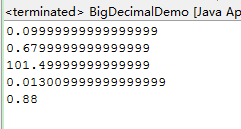float类型和double很容易丢失精度，所以我们试试Java为我们提供的BigDecimal类

• BigDecimal类成员方法
• public BigDecimal add(BigDecimal augend)：加
• public BigDecimal subtract(BigDecimal subtrahend)：减
• public BigDecimal multiply(BigDecimal multiplicand)：乘
• public BigDecimal divide(BigDecimal divisor)：除
• public BigDecimal divide(BigDecimal divisor,int scale,
int roundingMode) ：商，保留几位小数
public class BigDecimalDemo {
public static void main(String[] args) {

BigDecimal bd1 = new BigDecimal("0.09");
BigDecimal bd2 = new BigDecimal("0.01");

BigDecimal bd3 = new BigDecimal("1.0");
BigDecimal bd4 = new BigDecimal("0.32");
System.out.println("subtract:" + bd3.subtract(bd4));

BigDecimal bd5 = new BigDecimal("1.015");
BigDecimal bd6 = new BigDecimal("100");
System.out.println("multiply:" + bd5.multiply(bd6));

BigDecimal bd7 = new BigDecimal("1.301");
BigDecimal bd8 = new BigDecimal("100");
System.out.println("divide:" + bd7.divide(bd8));
System.out.println("divide:"+ bd7.divide(bd8, 3, BigDecimal.ROUND_HALF_UP));
System.out.println("divide:"+ bd7.divide(bd8, 8, BigDecimal.ROUND_HALF_UP));
}
}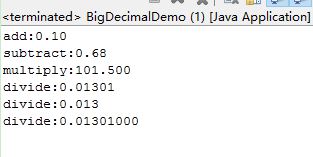• 下面我们来看看除法的详细说明：

• divide(BigDecimal divisor, int scale, introundingMode)
• BigDecimal的setScale方法 ：BigDecimal.setScale() 方法用于格式化小数点 ，表示保留一位小数，默认用四舍五入方式

• 直接删除多余的小数位，如2.35会变成2.3 setScale(1,BigDecimal.ROUND_DOWN)
• 进位处理，2.35变成2.4 setScale(1,BigDecimal.ROUND_UP)
• 四舍五入，2.35变成2.4 setScale(1,BigDecimal.ROUND_HALF_UP)
• 四舍五入，2.35变成2.3，如果是5则向下舍setScaler(1,BigDecimal.ROUND_HALF_DOWN)
• BigDecimal枚举常量用法摘要 ：
• CEILING 向正无限大方向舍入的舍入模式。
• DOWN 向零方向舍入的舍入模式。
• FLOOR 向负无限大方向舍入的舍入模式。
• HALF_DOWN 向最接近数字方向舍入的舍入模式，如果与两个相邻数字的距离相等，则向下舍入。
• HALF_EVEN 向最接近数字方向舍入的舍入模式，如果与两个相邻数字的距离相等，则向相邻的偶数舍入。
• HALF_UP 向最接近数字方向舍入的舍入模式，如果与两个相邻数字的距离相等，则向上舍入。
• UNNECESSARY 用于断言请求的操作具有精确结果的舍入模式，因此不需要舍入。
• UP 远离零方向舍入的舍入模式。

## Date类

• Date类概述
• 类 Date 表示特定的瞬间，精确到毫秒。
• 构造方法
• public Date():根据当前的默认毫秒值创建日期对象
• public Date(long date)：根据给定的毫秒值创建日期对象
public class DateDemo {
public static void main(String[] args) {
// 创建对象
Date d = new Date();
System.out.println("d:" + d)
//long time = System.currentTimeMillis();//获取当前的时间

// 创建对象
long time = 1000 * 60 * 60; // 1小时
Date d2 = new Date(time);
System.out.println("d2:" + d2);
}
}

• 成员方法
• public long getTime():获取时间，以毫秒为单位（从Date得到一个毫秒值）
• public void setTime(long time):设置时间（把一个毫秒值转换为Date）
public class DateDemo {
public static void main(String[] args) {
// 创建对象
Date d = new Date();
// 获取时间
long time = d.getTime();
System.out.println(time);
System.out.println(System.currentTimeMillis());
//运行结果可以发现，上面的结果是相同的

// 设置时间
d.setTime(1000);
System.out.println("d:" + d);
}
}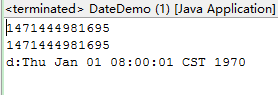## DateFormat类

• DateFormat类概述
• DateFormat 是日期/时间格式化子类的抽象类，它以与语言无关的方式格式化并解析日期或时间。
• 是抽象类，所以使用其子类SimpleDateFormat
• SimpleDateFormat构造方法

• public SimpleDateFormat():默认模式
• public SimpleDateFormat(String pattern):给定的模式

给定模式字符串该如何写呢?我们可以去看API找到对应的模式

• 年 y
• 月 M
• 日 d
• 时 H
• 分 m
• 秒 s
所以一般我们要这样书写”yyyy年MM月dd日 HH:mm:ss”
就会得到相应的日期：比如 2016年08月17日 23:05:16
• 成员方法

• public final String format(Date date)：Date – String(格式化)
• public Date parse(String source)：String – Date(解析)
• public class DateFormatDemo {
public static void main(String[] args) throws ParseException {
// Date -- String
// 创建日期对象
Date d = new Date();
// 创建格式化对象
//       SimpleDateFormat sdf = new SimpleDateFormat();
// 给定模式
SimpleDateFormat sdf = new SimpleDateFormat("yyyy年MM月dd日 HH:mm:ss");
// Date  --  String(格式化)
String s = sdf.format(d);
System.out.println(s);

//String -- Date(解析)
String str = "2016-08-08 12:12:12";
//在把一个字符串解析为日期的时候，请注意格式必须和给定的字符串格式匹配
SimpleDateFormat sdf2 = new SimpleDateFormat("yyyy-MM-dd HH:mm:ss");
Date dd = sdf2.parse(str);
System.out.println(dd);
}
}

输出结果：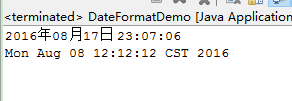我们来做一道不可思议的练习题，算一下你来到这个世界多少天?
纳尼，多少天，真不敢去想象啊！还是算一下吧。

public class MyYearOldDemo {
public static void main(String[] args) throws ParseException {
// 键盘录入你的出生的年月日，注意和下面的格式相同yyyy-MM-dd
Scanner sc = new Scanner(System.in);
System.out.println("请输入你的出生年月日:");
String line = sc.nextLine();

// 把该字符串转换为一个日期
SimpleDateFormat sdf = new SimpleDateFormat("yyyy-MM-dd");
Date d = sdf.parse(line);

// 通过该日期得到一个毫秒值
long myTime = d.getTime();

// 获取当前时间的毫秒值
long nowTime = System.currentTimeMillis();

// 用nowTime - myTime 得到一个毫秒值
long time = nowTime - myTime;

// 把time 的毫秒值转换为年
long day = time / 1000 / 60 / 60 / 24;

System.out.println("你来到这个世界：" + day + "天");
}
}

以我的出生年月日为例，输出结果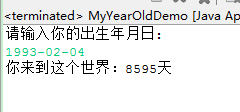8000多天，真的是老了

### Calendar类

• Calendar类概述
• Calendar 类是一个抽象类，它为特定瞬间与一组诸如 YEAR、MONTH、DAY_OF_MONTH、HOUR 等 日历字段之间的转换提供了一些方法，并为操作日历字段（例如获得下星期的日期）提供了一些方法。
• 成员方法
• public static Calendar getInstance()
• public int get(int field)：返回给定日历字段的值。日历类中的每个日历字段都是静态的成员变量，并且是int类型。
• public void add(int field,int amount)：根据给定的日历字段和对应的时间，来对当前的日历进行操作。
• public final void set(int year,int month,int date):设置当前日历的年月日
public class CalendarDemo {
public static void main(String[] args) {
// 其日历字段已由当前日期和时间初始化：
Calendar rightNow = Calendar.getInstance(); // 子类对象

// 获取年
int year = rightNow.get(Calendar.YEAR);
// 获取月
int month = rightNow.get(Calendar.MONTH);
// 获取日
int date = rightNow.get(Calendar.DATE);

System.out.println(year + "年" + (month + 1) + "月" + date + "日");
// 5年后的10天前
// 获取年
year = c.get(Calendar.YEAR);
// 获取月
month = c.get(Calendar.MONTH);
// 获取日
date = c.get(Calendar.DATE);
System.out.println(year + "年" + (month + 1) + "月" + date + "日");

c.set(2011, 11, 11);
// 获取年
year = c.get(Calendar.YEAR);
// 获取月
month = c.get(Calendar.MONTH);
// 获取日
date = c.get(Calendar.DATE);
System.out.println(year + "年" + (month + 1) + "月" + date + "日");
}
}

输出结果：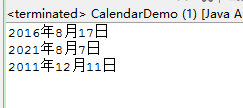到这里呢！我们的java中的常用类就学完了，不知道你掌握的怎么样呢？有时间多练习一下吧。我们下次开始学习集合。
欢迎有兴趣的同学加我朋友的QQ群：点击直接加群555974449 请备注：java基础再回首
我在群里等你。

还能输入1000个字符没有更多推荐了，返回首页